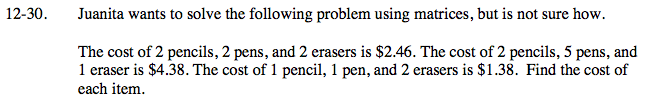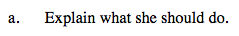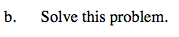### Home > PCT > Chapter Ch12 > Lesson 12.1.3 > Problem12-30

12-30.
1. Juanita wants to solve the following problem using matrices, but is not sure how. Homework Help ✎

2. The cost of 2 pencils, 2 pens, and 2 erasers is $2.46. The cost of 2 pencils, 5 pens, and 1 eraser is$4.38. The cost of 1 pencil, 1 pen, and 2 erasers is \$1.38. Find the cost of each item.

1. Explain what she should do.

2. Solve this problem.Think about how you would write an equation for each sentence.
What would your system of equations look like?